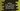# *args and **kwargs in Python and difference between them### *args and **kwargs in python :

In this python tutorial, we will discuss about two frequently used argument keywords ’args’ and ‘**kwargs’ . One thing we should keep in mind that we can use any name instead of ‘args’ and ‘kwargs’ for these variables. That means you can use any name like ’myargs’ , ‘**mykwargs’ etc. Let me show you what are the purpose of these two variables :

### What is *args:

*args is used to pass multiple argument values to a function. For example , following method is used to find the average of two numbers :

``````def find_avg(a,b):
average = (a+b)/2
print ("average is ",average)

find_avg(2,3)``````

We can pass any two number and it will print out the average result. But if you want to find average of 3 numbers, do you want to write a different method for it ? Again for 4 numbers ? Ofcourse we don’t want same styled method for each cases. What we want is we will pass any number of numbers to the same method and it will calculate the average. To achieve this, we can use ‘*args’ that allows to pass any number of arguments to a function. Following example shows how :

``````def find_avg(*numbers):
sum = 0
for i in numbers :
sum += i

print ("average is ",sum/(len(numbers)))
print (numbers)

find_avg(2,3)
find_avg(2,3,4)
find_avg(1,2,3,4,5,6,7,8,9,10)``````

It will give the following output :

``````average is  2.5
(2, 3)
average is  3.0
(2, 3, 4)
average is  5.5
(1, 2, 3, 4, 5, 6, 7, 8, 9, 10)``````

That means, we can pass any number of arguments if we place a ’’ before an argument name.Also , the second print statement shows that the values are stored as ‘tuple’ in the argument ’numbers’. Now what will happen if we place ’**’ ?

### What is **kwargs :

So, ‘*args’ is used to pass variable arguments to a function. Similarly, ‘**kwargs’ is used to pass variable ‘key-value’ arguments to a function. Example :

``````def print_values(**values):
print (values)

print_values(one = 1, two = 2)``````

If you run this program, it will print :

``{'one': 1, 'two': 2}``

That means, it passes a dictionary as an argument. As it is a dictionary, all dictionary operations can be performed. We can pass any number of key-value pairs :

``````def print_values(**values):
for key, value in values.items():
print("{} = {}".format(key,value))

print_values(one = 1,two = 2,three = 3,four = 4,five = 5)``````

Output :

``````one = 1
two = 2
three = 3
four = 4
five = 5``````

### Example to use *args and **kwargs in Function Calls :

We have seen that both ’args’ and ‘**kwargs’ are used to receive variable number of arguments in a function .Similarly, we can also put different number of arguments in ’args’ or ‘*kwargs’ and pass them to a different function. Previously, we have seen that if ’args’ is used in function definition, parameters are converted to a tuple and save it in ‘args’. So, to pass ‘*args’ as parameter, we need to assign it as a tuple of items.Example :

``````def avg_of_two(a,b):
print ((a+b)/2)

def avg_of_three(a,b,c):
print ((a+b+c)/3)

var1 = (1,2)
avg_of_two(*var1)

var2 = (1,2,3)
avg_of_three(*var2)``````

In this example, we have put some numbers in a tuple and assign it to a variable ‘var1’ and ‘var2’ . Next, we have passed the variable with a ’*’ before it. That’s it. The function definition handles all. e.g. after passing ‘var2’ to ‘avgofthree’ funciton, it assigns automatically 1 to ‘a’, 2 to ‘b’ and 3 to ‘c’.

Same thing can be achieved for ’kwargs’ as well. For ‘*args’, we were passing one tuple to the function . Now for ’kwargs’, we will pass one dictionary.Example :

``````def avg_of_two(a,b):
print ((a+b)/2)

def avg_of_three(a,b,c):
print ((a+b+c)/3)

var1 = {'a':1,'b':2}
avg_of_two(**var1)

var2 = {'a':1,'b':2,'c':3}
avg_of_three(**var2)``````

The output is same as above example.

### Using *args , **kwargs and other variables :

We can use *args , **kwargs and other normal variables in a same function. Example :

``````def show_details(a,b,*args,**kwargs):
print("a is ",a)
print("b is ",b)
print("args is ",args)
print("kwargs is ",kwargs)

show_details(1,2,3,4,5,6,7,8,9)
print("-----------")
show_details(1,2,3,4,5,6,c= 7,d = 8,e = 9)
print("-----------")``````

The output is :

``````a is  1
b is  2
args is  (3, 4, 5, 6, 7, 8, 9)
kwargs is  {}
-----------
a is  1
b is  2
args is  (3, 4, 5, 6)
kwargs is  {'c': 7, 'd': 8, 'e': 9}``````

That’s all for *args and **kwargs in python. Please drop a comment below and follow us on facebook or twitter :)